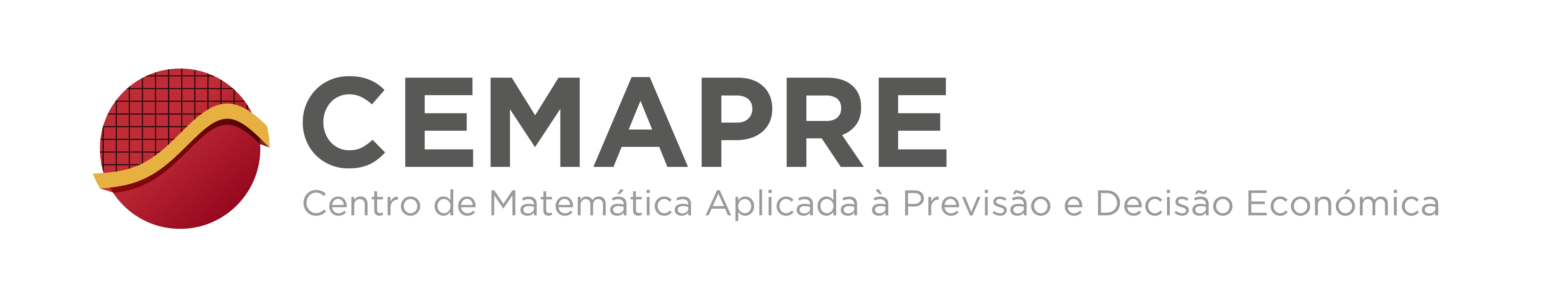## DYNAMICAL SYSTEMS Seminar

### Symbolic dynamics of piecewise contractions

Benito Pires
Abstract: A map $f:[0, 1] \to [0, 1]$ is a piecewise contraction if locally $f$ contracts distance, i.e., if there exist $0 <\lambda < 1$ and a partition of $[0, 1]$ into intervals $I_1, I_2, \ldots , I_n$ such that $\vert f(x) - f(y) \vert \leq \lambda \vert x - y \vert$ for all $x, y \in I_i$ ($1 \leq i \leq n$). Piecewise contractions describe the dynamics of many systems such as traffic control systems, queuing systems, outer billiards and Cherry flows. Here I am interested in the symbolic dynamics of such maps. More precisely, we say that an infinite word $i_0 i_1 i_2 \ldots$ over the alphabet $\mathcal{A} = \{1, 2, \vert , n\}$ is the natural coding of $x \in [0, 1]$ if $f^k(x) \in I_{i_k}$ for all $k \geq 0$. The aim of this talk is to provide a complete classification of the words that appear as natural codings of injective piecewise contractions.